# Java Program for Addition, Subtraction, Multiplication, and Division

In this example, you'll learn how to perform addition, subtraction, multiplication, and division of any two numbers in Java. Before starting the actual program, let's first create a very simple program that performs four basic mathematical operations without user input.

## Addition, Subtraction, Multiplication, and Division without User Input

The question is: write a Java program that performs four basic mathematical operations such as addition, subtraction, multiplication, and division. Here is its answer: I've not allowed the user to feed the input for this program, but later on, this program will be modified to allow the user to provide the input at run-time of the program to perform operations on two desired numbers.

```public class CodesCracker
{
public static void main(String[] args)
{
int num1 = 20, num2 = 15, res;

res = num1 + num2;
System.out.println("Addition Result = " + res);
res = num1 - num2;
System.out.println("Subtraction Result = " + res);
res = num1 * num2;
System.out.println("Multiplication Result = " + res);
res = num1 / num2;
System.out.println("Division Result = " + res);
}
}```

This Java program will exactly produce the following output: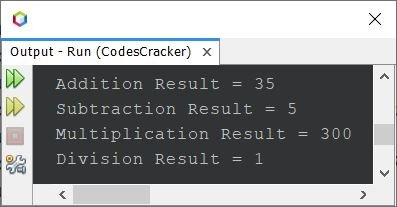## Addition, Subtraction, Multiplication, and Division with User Input

This program is basically a modified version of the previous program, as it allows the user to enter numbers for the program. The rest of the things are similar to the previous program.

```import java.util.Scanner;

public class CodesCracker
{
public static void main(String[] args)
{
Scanner scan = new Scanner(System.in);
int num1, num2, res;

System.out.println("Enter the First Number: ");
num1 = scan.nextInt();
System.out.println("Enter the Second Number: ");
num2 = scan.nextInt();

res = num1 + num2;
System.out.println("\nAddition Result = " + res);
res = num1 - num2;
System.out.println("Subtraction Result = " + res);
res = num1 * num2;
System.out.println("Multiplication Result = " + res);
res = num1 / num2;
System.out.println("Division Result = " + res);
}
}```

Here is its sample run with user input of 44 as the first number and 23 as the second number: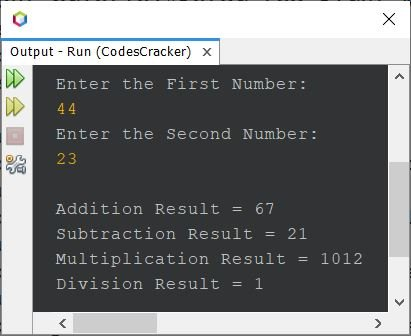## Basic Mathematical Operation in Java: Complete Version

This is the complete version of the above program, as it handles errors too. Sometimes, users enter wrong or invalid inputs like c or # in place of numbers. Therefore, the program must be created in a way to handle those types of errors.

```import java.util.Scanner;
import java.util.InputMismatchException;

public class CodesCracker
{
public static void main(String[] args)
{
Scanner scan = new Scanner(System.in);
double num1, num2, res;

System.out.println("Enter the First Number: ");
try
{
num1 = scan.nextDouble();
}
catch(InputMismatchException e)
{
System.out.println("\nInvalid Input!");
System.out.println("Exception Name: " + e);
return;
}

System.out.println("Enter the Second Number: ");
try
{
num2 = scan.nextDouble();
}
catch(InputMismatchException e)
{
System.out.println("\nInvalid Input!");
System.out.println("Exception Name: " + e);
return;
}

res = num1 + num2;
System.out.println("\nAddition Result = " + res);
res = num1 - num2;
System.out.println("Subtraction Result = " + res);
res = num1 * num2;
System.out.println("Multiplication Result = " + res);
res = num1 / num2;
System.out.println("Division Result = " + res);
}
}```

Here is its sample run with user input of 32 as the first number and 31 as the second number: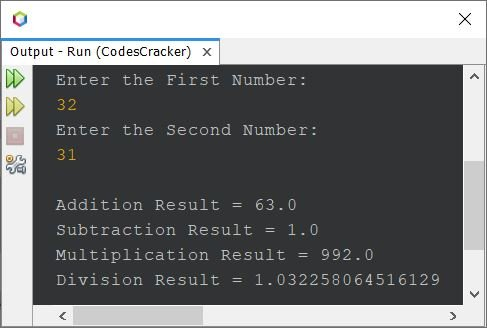Here is another sample run with an invalid user input as the second number, say 32 as the first number but c as the second number: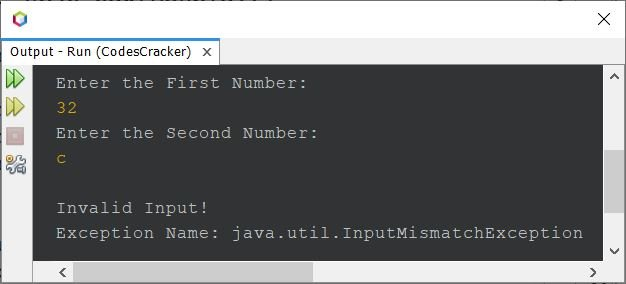If you enter any invalid input as the first number, then the program prints an error message saying "Invalid Input!" along with the exception name. Also, the second number will not get received from the user by the program because I've used the "return" keyword to stop the remaining execution of the program when the user feeds an invalid input. For example, let's take another sample run with user input "codescracker" as the first number: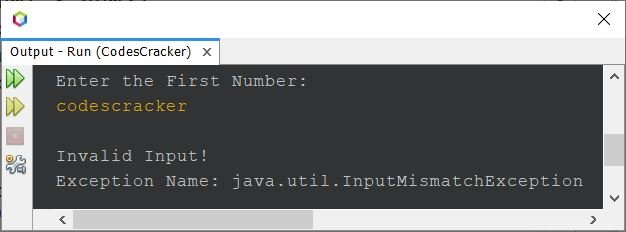## Add, Subtract, Multiply, and divide based on the user's Choice

This is the minimal version of the above program, as it performs only one desired operation at a time based on the user's choice.

```import java.util.Scanner;

public class CodesCracker
{
public static void main(String[] args)
{
Scanner scan = new Scanner(System.in);
float num1, num2, res;
char ch;

System.out.println("Enter any Two Numbers: ");
num1 = scan.nextFloat();
num2 = scan.nextFloat();
System.out.println("Enter the Operator (+, -, *, /): ");
ch = scan.next().charAt(0);
if(ch == '+') res = num1 + num2;
else if(ch == '-') res = num1 - num2;
else if(ch == '*') res = num1 * num2;
else if(ch == '/') res = num1 / num2;
else
{
System.out.println("\nInvalid Input");
return;
}
System.out.println("\nResult = " + res);
}
}```

Here is its sample run with user input of 10 and 30 as the first and second numbers and + as the operator to perform the addition of these two numbers only: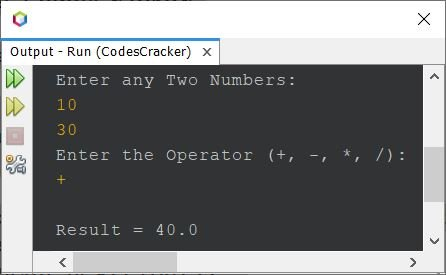The above program uses if...else if...else conditional statements to check and perform the operation based on the operator entered by the user.

#### Same Program in Other Languages

Java Online Test

« Previous Program Next Program »